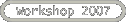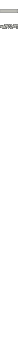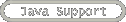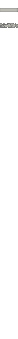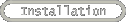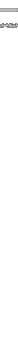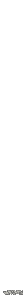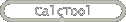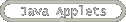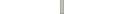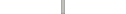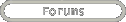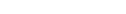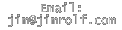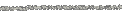A method is a subroutine that does something.  For example, we may want to define and evaluate a function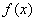.  We will utilize a method to evaluate this function and return the answer to a user-specified location.

Methods must be declared in the main body of your program.

This means they will never be declared in your computeButtonMouseClicked()method, nor in any other method.

In general, methods have the form

<return type> methodName(<type> argument 1, <type> argument 2, …){
//code goes here
return answer of type <return type>;
}

<return type> can be any type of variable or object, such as double, int , boolean , etc.  You may even have a return type of void .  This means that the method does something, but returns nothing.  In the case of a void method, the last line,

return answer of type <return type>;

cannot be used.

Here’s an example of a method that calculates.  In order to do this, we will need to input x as a double and return the answer as a double.  So the method might look like:

double f(double x){
double answer=Math.sin(x)*Math.sin(x);    //note the use of the
// Math class here.
// It has lots of functions!
}

If we wanted to calculate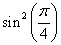in the context of our computeButton method and put the result in the variable funcAnswer we would do this by

Some other Math functions and constants can be found here.

• Add a method named sum  to your code that returns the value of the sum of the integers 1 through n.  The sum of the first n integers can be computed via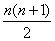.  You have a couple of options to implement this method.  Your may want to write a method that returns a double value.  But you can also take advantage of the fact that this method always returns an integer, so you might choose a return type of int.  Also the argument of this method can be an double or an int as well.    So you have two reasonable choices for the argument type.  You should pay special attention when dividing by two ints.    For example, 3/2 is 1, not 1.5.  1/4 is 0, not 0.25.

See Sample Code 3 to check work

• Test your method by outputting to the methodLabel.  Your applet should now take an input n, and return the sum of the first n integers computed two different ways—via a loop and via a method.  Build and run your project.